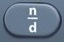# Mean

Mean =$\bf\displaystyle\frac{Sum}{Count}$

Basics

The term mean is synonymous with the term average.

The formula provided for mean by the Mathematics Formula Sheet is not presented as a mathematical expression.

Formulas (Mathematics Formula Sheet)

Mean is equal to the total of the values of a data set, divided by the number of elements in the data set.

Formulas (NOT on Mathematics Formula Sheet)
Mean =$\bf\displaystyle\frac{Sum}{Count}$

Sum = Mean x Count

Count =$\bf\displaystyle \frac{Sum}{Mean}$

Count = number of values

Question
Three people weigh 170, 186, and 244 pounds, respectively.  What is their mean weight?

200 pounds

Sum = addition of values = 170 + 186 + 244
Count = number of values = 3

Mean =$\bf\displaystyle\frac{Sum}{Count}$

Mean =$\bf\displaystyle\frac{170 + 186 + 244}{3}$ = 200

Input Display Commentblinker clears screen170+186+2443$\bf\displaystyle\frac{170+186+244}{3}$ Mean =$\bf\displaystyle\frac{Sum}{Count}$200 Answer
###### Mean

Question
Five people weigh 120, 150, 170, 186, and 244 pounds, respectively.  What is their average weight?

174 pounds

Average is the same as Mean.

Sum = addition of values = 120 + 150 + 170 + 186 + 244
Count = number of values = 5

Mean =$\bf\displaystyle\frac{Sum}{Count}$

Mean =$\bf\displaystyle\frac{120 + 150 + 170 + 186 + 244}{5}$ = 174

Input Display Commentblinker clears screen120+150+170+186+244$\bf\displaystyle\frac{120 + 150 + 170 + 186 + 244}{5}$ Mean =$\bf\displaystyle\frac{Sum}{Count}$174 Answer
###### Mean

Question

The mean weight of four people is 165 pounds.  What is their total weight?

660 pounds

Sum = Mean x Count
Sum = 165 × 4 = 660

Input Display Commentblinker clears screen
165 × 4 165*4 Sum = Mean x Count660 Answer
###### Mean

Question

A group of people with a mean weight of 135 pounds weigh a total of 945 pounds.  How many people are in the group?

7

Count =$\bf\displaystyle \frac{Sum}{Mean}$

Count =$\bf\displaystyle \frac{945}{135}$ = 7

Input Display Commentblinker clears screen945135$\bf\displaystyle \frac{945}{135}$ Count =$\bf\displaystyle \frac{Sum}{Mean}$7 Answer
###### Mean

Question

Three people have a mean weight of 145 pounds.  One person weighs 132 pounds.  A second person weighs 186 pounds.  What does the third person weigh?

117 pounds

Sum = Mean × Count
Sum = Mean × Count = 145 × 3 = 435

Unknown Value = Sum – Known Values
Unknown Value = 435 – 132 – 186 = 117

Input Display Commentblinker clears screen
145 × 3 145*3 Sum = Mean × Count435 Sum
435 – 132 – 186 435-132-186 Unknown Value =
Sum – Known Values117 Answer
###### Mean

Practice – Questions
1.  Five basketball players scored 7, 12, 15, 28, and 33 points, respectively.  What was their mean score?

2.  Five basketball players scored 3, 6, 11, 18, and 47 points, respectively.  What was their average score?

3.  The mean bowling score of 8 people was 133.  What was their total score?

4.  A group of bowlers with a mean score of 147 had a total of score of 1617.  How many bowlers were in the group?

5.  Four bowlers had a mean score of 168.  One bowler scored 111.  A second bowler scored 133.  A third bowler scored 223.  What did the fourth bowler score?